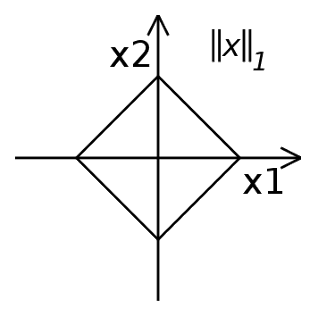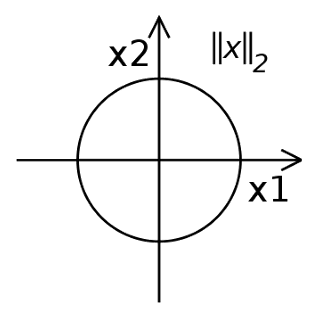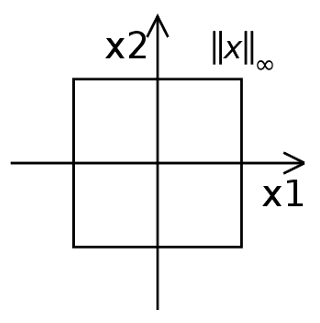# Sabbiu Shah

## p-Norms (Taxicab Norm, Euclidean Norm and infinity-norm)

Norms are a measure of distance. Norm is defined as follows: For $p\geq1$,

$||x||_p \equiv \sqrt[p]{|x_1|^p + |x_2|^p + ... + |x_n|^p}$

### Taxicab Norm (1-Norm)

When $p=1$, then the norm is said to be taxicab norm. The distance derived from this norm is called Manhattan distance.

$||x||_1 \equiv |x_1| + |x_2| + ... + |x_n|$

### Euclidean Norm (2-Norm)

It is the most common notion of distance. When $p=2$, then the norm is said to be euclidean norm.

$||x||_2 \equiv \sqrt{|x_1|^2 + |x_2|^2 + ... + |x_n|^2}$

### ∞-norm

Infinity norm is defined as,

$||x||_\infty \equiv max(|x_1|, |x_2|, ..., |x_n|)$

Proof

\begin{aligned} ||x||_p & \equiv \sum_{i=1}^n |x_i|^p\ \ \ \ \ \ \ \ \ \ \text{Equation of p-norm}\\ ||x||_p & \equiv m \sum_{i=1}^n \frac{|x_i|^p}{m}\ \ \ \ \ \ \ \ \ \ m=max(|x_i|)\\ \end{aligned}

As, $p$ approaches $\infty$, only the term $\frac{max|x_i|}{m}$ equals to $1$, while other terms approaches to $0$. Thus, $\sum_{i=1}^n \frac{|x_i|^p}{m} = 1$

\begin{aligned} \therefore\ ||x||_\infty & \equiv max(|x_i|) \end{aligned}

### Visualising norms as a unit circle

This section will show visualization when, $||x||_p \equiv 1$. Let us consider for 2 Dimensional case.

#### 1-Norm

The equation is given as,

\begin{aligned} & ||x||_1 = |x_1| + |x_2|\\ \implies & 1= |x_1| + |x_2|\\ \end{aligned}

Thus we get the following equations,
When $x_1\geq0$ and $x_2\geq0$, $x_2=1-x_1$ [First quadrant]
When $x_1\leq0$ and $x_2\geq0$, $x_2=1+x_1$ [Second quadrant]
When $x_1\leq0$ and $x_2\leq0$, $x_2=x_1-1$ [Third quadrant]
When $x_1\geq0$ and $x_2\leq0$, $x_2=-x_1-1$ [Fourth quadrant]

Plotting these equations, we get,#### 2-Norm

The equation is given as,

\begin{aligned} & ||x||_2 = \sqrt{|x_1|^2 + |x_2|^2}\\ \implies & 1= x_1^2 + x_2^2\\ \end{aligned}

As this equation represents a unit circle, we get the following graph,#### ∞-norm

The equation is given as,

\begin{aligned} & ||x||_\infty = max(|x_1|, |x_2|)\\ \implies & 1 = max(|x_1|, |x_2|)\\ \end{aligned}

This gives the following graph,Substituting different values of p, these equations can be further visualised in Wolfram Mathematica Demonstaration